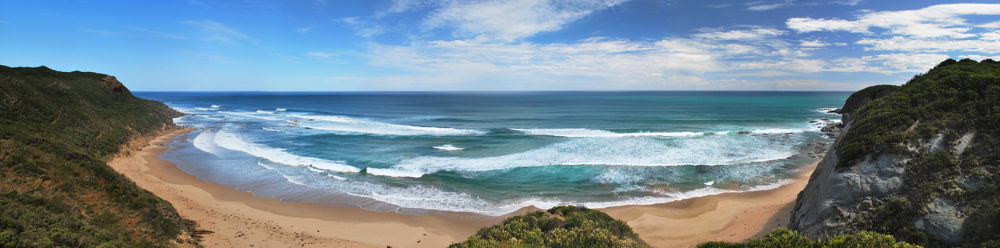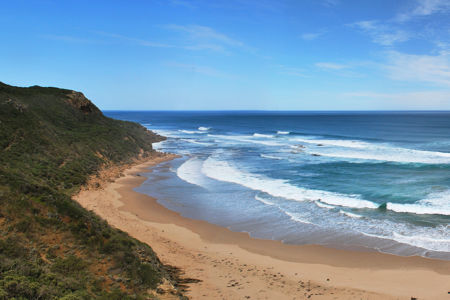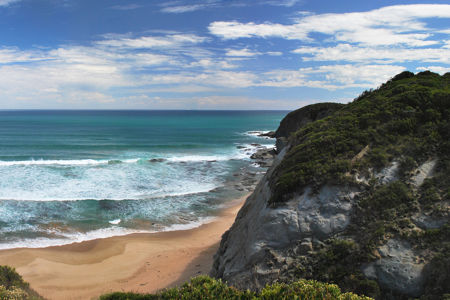Cloudimage - Documentation

## Focal point crop

Allows to crop an image by defining the coordinates of the crop focal point. It is useful when you want to crop very tall or very wide images where the coordinates of the point of interest are already known.

To set the coordinates of the focal point, use the gravity=X,Y operation where X and Y are the coordinates of the focal point (in pixels). Alternatively, you can set the coordinates as a percentage of the original image - gravity=Xp,Yp or of the resized image - gravity=Xs,Ys where X,Y=0...100 (decimals are accepted) or gravity=xc,yc, where x,y=0..1 (the provided number is multiplied by 100, with maximum value 100).

gravity=X,Y

gravity=Xp|Xs|xc,Yp|Ys|yc

### Example

#### Original image

/sample.li/castle.jpg?w=1000#### Cropped image, focus X=1700

/sample.li/castle.jpg?func=crop&w=450&h=300&gravity=1750,1000#### Cropped image, focus X=80%

/sample.li/castle.jpg?func=crop&w=450&h=300&gravity=80p,50pPlease note that the focal point in gravity=X,Y has to be defined as coordinates X and Y of the full-sized origin image and not the scaled down version.